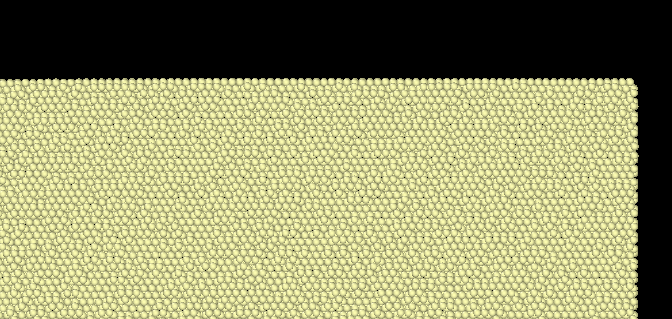# Regarding periodicity of Beryllium along Z-dimesion

Dear Lammps Users,

I am simulating Be system of particles whose lattice is described below(HCP). Z-dimension is kept in periodic. As a rule if any dimension is in periodicity, it should be defined in such a way that it should be in integral multiple of the lattice constant in that dimension.
Although I am using a multiple of ‘variable c’ (28 times of c in this case) but atoms are getting unstable at the boundaries.
Kindly suggest to make the periodicity work in Z-direction.

variable a equal 2.312646565
variable b equal \$a*sqrt(3.0)
variable c equal \$a*1.5682

lattice custom 1.0 a1 \$a 0.0 0.0&
a2 0.0 \$b 0.0&
a3 0.0 0.0 \$c&
basis 0.0 0.0 0.0&
basis 0.5 0.5 0.0&
basis 0.0 0.33333333 0.5&
basis 0.5 0.83333333 0.5

variable xi equal 0
variable xm equal 200
variable yi equal 0
variable ym equal 80
variable zi equal -50.77369281
variable zm equal 50.77369281

# Simulation box

region s_box block 0 350 0 200 (v_zi) (v_zm) units box

The atoms are shown in Figure as below. they are loosing their structure at the boundaries.Regards.

Dear Lammps Users,

I am simulating Be system of particles whose lattice is described below(HCP). Z-dimension is kept in periodic. As a rule if any dimension is in periodicity, it should be defined in such a way that it should be in integral multiple of the lattice constant in that dimension.
Although I am using a multiple of ‘variable c’ (28 times of c in this case) but atoms are getting unstable at the boundaries.
Kindly suggest to make the periodicity work in Z-direction.

your positions are ambiguous, because you put your box boundary exactly on the atom positions.
your manual computation is not accurate enough and thus you are subject to rounding errors.

as has been discussed here many times, the best way to deal with this and avoid ambiguity, is to shift the box by a small margin relative to the origin (or shift the lattice).

axel.